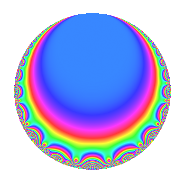Properties

 Label 6012.2.a.aLevel 6012 Weight 2 Character orbit 6012.a Self dual Yes Analytic conductor 48.006 Analytic rank 0 Dimension 2 CM No Inner twists 1

Related objects

Newspace parameters

 Level: $$N$$ = $$6012 = 2^{2} \cdot 3^{2} \cdot 167$$ Weight: $$k$$ = $$2$$ Character orbit: $$[\chi]$$ = 6012.a (trivial)

Newform invariants

 Self dual: Yes Analytic conductor: $$48.0060616952$$ Analytic rank: $$0$$ Dimension: $$2$$ Coefficient field: $$\Q(\sqrt{13})$$ Coefficient ring: $$\Z[a_1, \ldots, a_{7}]$$ Coefficient ring index: $$1$$ Fricke sign: $$-1$$ Sato-Tate group: $\mathrm{SU}(2)$

$q$-expansion

Coefficients of the $$q$$-expansion are expressed in terms of $$\beta = \frac{1}{2}(1 + \sqrt{13})$$. We also show the integral $$q$$-expansion of the trace form.

 $$f(q)$$ $$=$$ $$q$$ $$+ 3 q^{5}$$ $$+ ( 2 - \beta ) q^{7}$$ $$+O(q^{10})$$ $$q$$ $$+ 3 q^{5}$$ $$+ ( 2 - \beta ) q^{7}$$ $$+ ( 5 - \beta ) q^{13}$$ $$-\beta q^{17}$$ $$+ 2 q^{19}$$ $$+ \beta q^{23}$$ $$+ 4 q^{25}$$ $$+ ( -3 - 2 \beta ) q^{29}$$ $$+ ( 2 + 2 \beta ) q^{31}$$ $$+ ( 6 - 3 \beta ) q^{35}$$ $$+ ( 5 - 2 \beta ) q^{37}$$ $$+ ( -3 + 4 \beta ) q^{41}$$ $$+ ( 5 + 2 \beta ) q^{43}$$ $$+ ( 3 - 2 \beta ) q^{47}$$ $$-3 \beta q^{49}$$ $$+ 2 \beta q^{53}$$ $$+ ( 6 - 6 \beta ) q^{59}$$ $$+ ( -7 + 6 \beta ) q^{61}$$ $$+ ( 15 - 3 \beta ) q^{65}$$ $$+ ( -1 + 4 \beta ) q^{67}$$ $$+ ( 3 - 3 \beta ) q^{71}$$ $$+ ( -10 + 3 \beta ) q^{73}$$ $$+ ( 11 - 2 \beta ) q^{79}$$ $$+ ( -3 + 2 \beta ) q^{83}$$ $$-3 \beta q^{85}$$ $$+ 6 \beta q^{89}$$ $$+ ( 13 - 6 \beta ) q^{91}$$ $$+ 6 q^{95}$$ $$+ ( -7 + 5 \beta ) q^{97}$$ $$+O(q^{100})$$ $$\operatorname{Tr}(f)(q)$$ $$=$$ $$2q$$ $$\mathstrut +\mathstrut 6q^{5}$$ $$\mathstrut +\mathstrut 3q^{7}$$ $$\mathstrut +\mathstrut O(q^{10})$$ $$2q$$ $$\mathstrut +\mathstrut 6q^{5}$$ $$\mathstrut +\mathstrut 3q^{7}$$ $$\mathstrut +\mathstrut 9q^{13}$$ $$\mathstrut -\mathstrut q^{17}$$ $$\mathstrut +\mathstrut 4q^{19}$$ $$\mathstrut +\mathstrut q^{23}$$ $$\mathstrut +\mathstrut 8q^{25}$$ $$\mathstrut -\mathstrut 8q^{29}$$ $$\mathstrut +\mathstrut 6q^{31}$$ $$\mathstrut +\mathstrut 9q^{35}$$ $$\mathstrut +\mathstrut 8q^{37}$$ $$\mathstrut -\mathstrut 2q^{41}$$ $$\mathstrut +\mathstrut 12q^{43}$$ $$\mathstrut +\mathstrut 4q^{47}$$ $$\mathstrut -\mathstrut 3q^{49}$$ $$\mathstrut +\mathstrut 2q^{53}$$ $$\mathstrut +\mathstrut 6q^{59}$$ $$\mathstrut -\mathstrut 8q^{61}$$ $$\mathstrut +\mathstrut 27q^{65}$$ $$\mathstrut +\mathstrut 2q^{67}$$ $$\mathstrut +\mathstrut 3q^{71}$$ $$\mathstrut -\mathstrut 17q^{73}$$ $$\mathstrut +\mathstrut 20q^{79}$$ $$\mathstrut -\mathstrut 4q^{83}$$ $$\mathstrut -\mathstrut 3q^{85}$$ $$\mathstrut +\mathstrut 6q^{89}$$ $$\mathstrut +\mathstrut 20q^{91}$$ $$\mathstrut +\mathstrut 12q^{95}$$ $$\mathstrut -\mathstrut 9q^{97}$$ $$\mathstrut +\mathstrut O(q^{100})$$

Embeddings

For each embedding $$\iota_m$$ of the coefficient field, the values $$\iota_m(a_n)$$ are shown below.

For more information on an embedded modular form you can click on its label.

Label $$\iota_m(\nu)$$ $$a_{2}$$ $$a_{3}$$ $$a_{4}$$ $$a_{5}$$ $$a_{6}$$ $$a_{7}$$ $$a_{8}$$ $$a_{9}$$ $$a_{10}$$
1.1
 2.30278 −1.30278
0 0 0 3.00000 0 −0.302776 0 0 0
1.2 0 0 0 3.00000 0 3.30278 0 0 0
 $$n$$: e.g. 2-40 or 990-1000 Significant digits: Format: Complex embeddings Normalized embeddings Satake parameters Satake angles

Inner twists

This newform does not admit any (nontrivial) inner twists.

Atkin-Lehner signs

$$p$$ Sign
$$2$$ $$-1$$
$$3$$ $$-1$$
$$167$$ $$-1$$

Hecke kernels

This newform can be constructed as the kernel of the linear operator $$T_{5}$$ $$\mathstrut -\mathstrut 3$$ acting on $$S_{2}^{\mathrm{new}}(\Gamma_0(6012))$$.